# 顶点数据管理

• 顶点压缩
• 顶点流拆分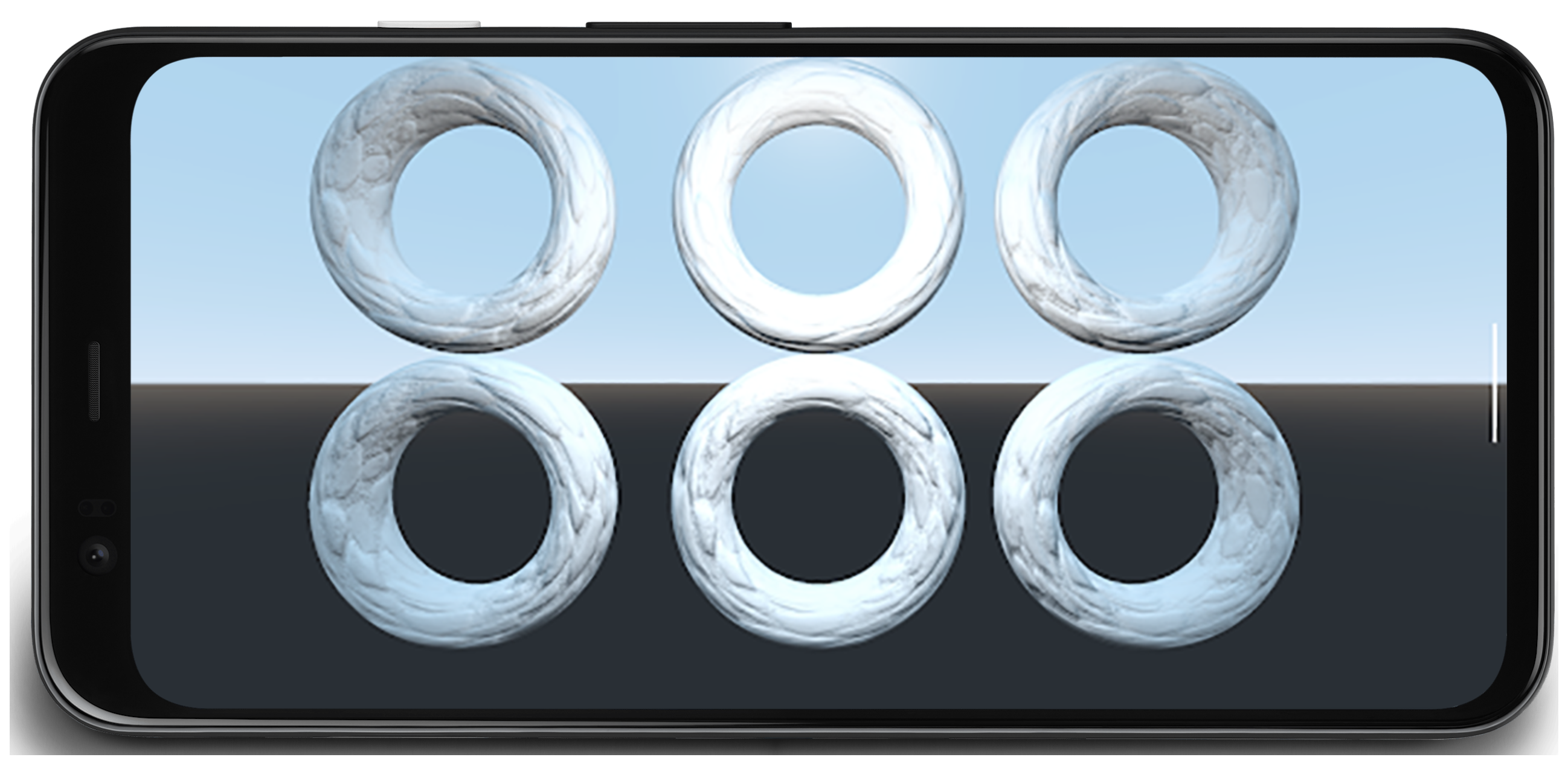## 顶点压缩

• 降低顶点数据属性的数值精度（例如从 32 位浮点数降低到 16 位浮点数）
• 以不同的格式表示属性

### 顶点位置

``````uint16_t f32_to_f16(float f) {
uint32_t x = (uint32_t)f;
uint32_t sign = (unsigned short)(x >> 31);
uint32_t mantissa;
uint32_t exp;
uint16_t hf;

mantissa = x & ((1 << 23) - 1);
exp = x & (0xFF << 23);
if (exp >= 0x47800000) {
// check if the original number is a NaN
if (mantissa && (exp == (0xFF << 23))) {
// single precision NaN
mantissa = (1 << 23) - 1;
} else {
// half-float will be Inf
mantissa = 0;
}
hf = (((uint16_t)sign) << 15) | (uint16_t)((0x1F << 10)) |
(uint16_t)(mantissa >> 13);
}
// check if exponent is <= -15
else if (exp <= 0x38000000) {
hf = 0;  // too small to be represented
} else {
hf = (((uint16_t)sign) << 15) | (uint16_t)((exp - 0x38000000) >> 13) |
(uint16_t)(mantissa >> 13);
}

return hf;
}
``````

``````for each position p in Mesh:
p -= center_of_bounding_box // Moves Mesh back to the center of model space
p /= half_size_bounding_box // Fits the mesh into a [-1, 1] cube
vec3<float16> result = vec3(f32_to_f16(p.x), f32_to_f16(p.y), f32_to_f16(p.z));
``````

``````vec3 in in_pos;

void main() {
...
// bounding box data packed into uniform buffer
vec3 decompress_pos = in_pos * half_size_bounding_box + center_of_bounding_box;
gl_Position = proj * view * model * decompress_pos;
}
``````

``````const int BITS = 16

for each position p in Mesh:
p -= center_of_bounding_box // Moves Mesh back to the center of model space
p /= half_size_bounding_box // Fits the mesh into a [-1, 1] cube
// float to integer value conversion
p = clamp(p * (2^(BITS - 1) - 1), -2^(BITS - 1), 2^(BITS - 1) - 1)
``````

### 顶点法线和切线空间

#### 切线空间

``````const int BITS = 16

quaternion tangent_space_to_quat(vec3 normal, vec3 tangent, vec3 bitangent) {
mat3 tbn = {normal, tangent, bitangent};
quaternion qTangent(tbn);
qTangent.normalize();

//Make sure QTangent is always positive
if (qTangent.w < 0)
qTangent = -qTangent;

const float bias = 1.0 / (2^(BITS - 1) - 1);

//we need to apply a "bias"; while making sure the Quaternion
//stays normalized.
// ** Also our shaders assume qTangent.w is never 0. **
if (qTangent.w < bias) {
Real normFactor = Math::Sqrt( 1 - bias * bias );
qTangent.w = bias;
qTangent.x *= normFactor;
qTangent.y *= normFactor;
qTangent.z *= normFactor;
}

//If it's reflected, then make sure .w is negative.
vec3 naturalBinormal = cross_product(tangent, normal);
if (dot_product(naturalBinormal, binormal) <= 0)
qTangent = -qTangent;
return qTangent;
}
``````

``````for each vertex v in mesh:
quaternion res = tangent_space_to_quat(v.normal, v.tangent, v.bitangent);
// Once we have the quaternion we can compress it
res = clamp(res * (2^(BITS - 1) - 1), -2^(BITS - 1), 2^(BITS - 1) - 1);
``````

``````vec3 xAxis( vec4 qQuat )
{
float fTy  = 2.0 * qQuat.y;
float fTz  = 2.0 * qQuat.z;
float fTwy = fTy * qQuat.w;
float fTwz = fTz * qQuat.w;
float fTxy = fTy * qQuat.x;
float fTxz = fTz * qQuat.x;
float fTyy = fTy * qQuat.y;
float fTzz = fTz * qQuat.z;

return vec3( 1.0-(fTyy+fTzz), fTxy+fTwz, fTxz-fTwy );
}

vec3 yAxis( vec4 qQuat )
{
float fTx  = 2.0 * qQuat.x;
float fTy  = 2.0 * qQuat.y;
float fTz  = 2.0 * qQuat.z;
float fTwx = fTx * qQuat.w;
float fTwz = fTz * qQuat.w;
float fTxx = fTx * qQuat.x;
float fTxy = fTy * qQuat.x;
float fTyz = fTz * qQuat.y;
float fTzz = fTz * qQuat.z;

return vec3( fTxy-fTwz, 1.0-(fTxx+fTzz), fTyz+fTwx );
}

void main() {
vec4 qtangent = normalize(in_qtangent); //Needed because 16-bit quantization
vec3 normal = xAxis(qtangent);
vec3 tangent = yAxis(qtangent);
float biNormalReflection = sign(in_qtangent.w); //ensured qtangent.w != 0
vec3 binormal = cross(normal, tangent) * biNormalReflection;
...
}
``````

#### 仅法线``````const int BITS = 8

// Assumes the vector is unit length
// sign() function should return positive for 0
for each normal n in mesh:
float invL1Norm = 1.0 / (abs(n.x) + abs(n.y) + abs(n.z));
vec2 res;
if (n.z < 0.0) {
res.x = (1.0 - abs(n.y * invL1Norm)) * sign(n.x);
res.y = (1.0 - abs(n.x * invL1Norm)) * sign(n.y);
} else {
res.x = n.x * invL1Norm;
res.y = n.y * invL1Norm;
}
res = clamp(res * (2^(BITS - 1) - 1), -2^(BITS - 1), 2^(BITS - 1) - 1)
``````

``````//Additional Optimization: twitter.com/Stubbesaurus/status/937994790553227264
vec3 oct_to_vec(vec2 e):
vec3 v = vec3(e.xy, 1.0 - abs(e.x) - abs(e.y));
float t = max(-v.z, 0.0);
v.xy += t * -sign(v.xy);
return v;
``````

``````const int BITS = 8
const float bias = 1.0 / (2^(BITS - 1) - 1)

// Compressing
for each normal n in mesh:
//encode to octahedron, result in range [-1, 1]
vec2 res = vec_to_oct(n);

// map y to always be positive
res.y = res.y * 0.5 + 0.5;

if (res.y < bias)
res.y = bias;

// Apply the sign of the binormal to y, which was computed elsewhere
if (binormal_sign < 0)
res.y *= -1;

res = clamp(res * (2^(BITS - 1) - 1), -2^(BITS - 1), 2^(BITS - 1) - 1)
``````
``````// Vertex shader decompression
vec2 encode = vec2(tangent_encoded.x, abs(tangent_encoded.y) * 2.0 - 1.0));
vec3 tangent_real = oct_to_vec3(encode);
float binormal_sign = sign(tangent_encode.y);
``````

### 顶点 UV 坐标

``````const int BITS = 16

for each vertex_uv V in mesh:
V *= clamp(2^BITS - 1, 0, 2^BITS - 1);  // float to integer value conversion
``````

### 顶点压缩结果

• 顶点内存读取带宽：
• 分箱：27GB/s 到 9GB/s
• 渲染：4.5GB/s 到 1.5GB/s
• 顶点提取暂停：
• 分箱：50% 到 0%
• 渲染：90% 到 90%
• 平均字节/顶点数：
• 分箱：48 字节到 16 字节
• 渲染：52 字节到 18 字节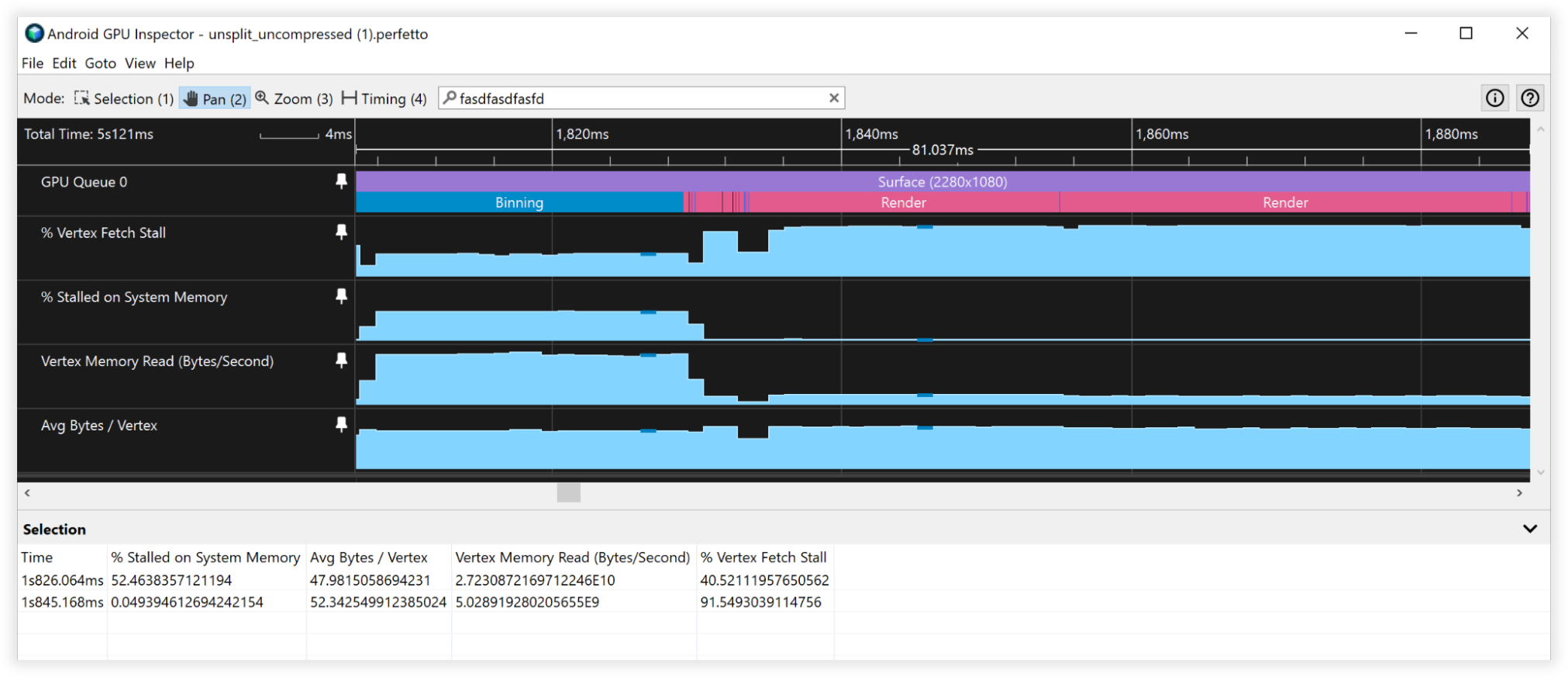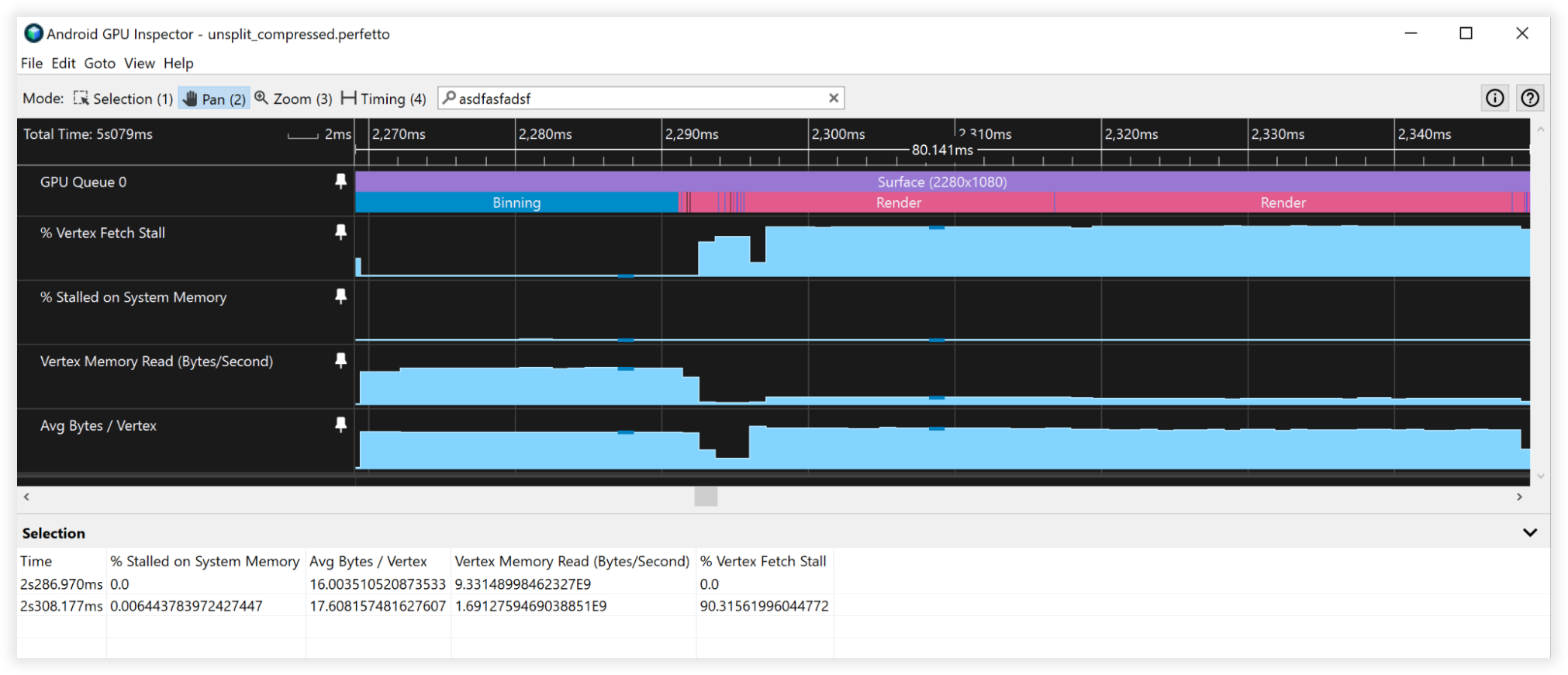## 顶点流拆分

``````Before:
|Position1/Normal1/Tangent1/UV1/Position2/Normal2/Tangent2/UV2......|

After:
|Position1/Position2...|Normal1/Tangent1/UV1/Normal2/Tangent2/UV2...|
``````

• 32 字节的缓存行（相当常见的大小）
• 顶点格式由以下内容组成：
• 位置 vec3<float32> = 12 字节
• 法线 vec3<float32> = 12 字节
• UV 坐标 vec2<float32> = 8 字节
• 总大小 = 32 字节

### 顶点流拆分结果

• 顶点内存读取带宽：
• 分箱：27GB/s 到 6.5GB/s
• 渲染：4.5GB/s 到 4.5GB/s
• 顶点提取暂停：
• 分箱：40% 到 0%
• 渲染：90% 到 90%
• 平均字节/顶点数：
• 分箱：48 字节到 12 字节
• 渲染：52 字节到 52 字节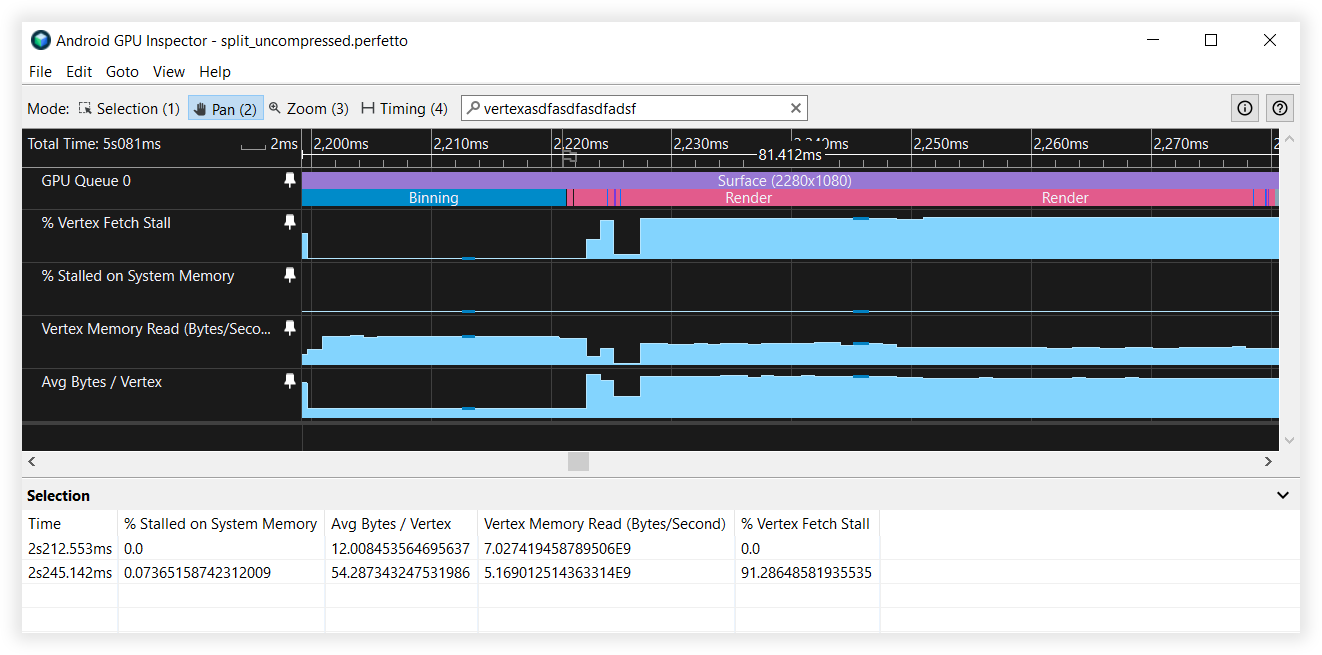## 合用结果

• 顶点内存读取带宽：
• 分箱：25GB/s 到4.5 GB/s
• 渲染：4.5GB/s 到 1.7GB/s
• 顶点提取暂停：
• 分箱：41% 到 0%
• 渲染：90% 到 90%
• 平均字节/顶点数：
• 分箱：48 字节到 8 字节
• 渲染：52 字节到 19 字节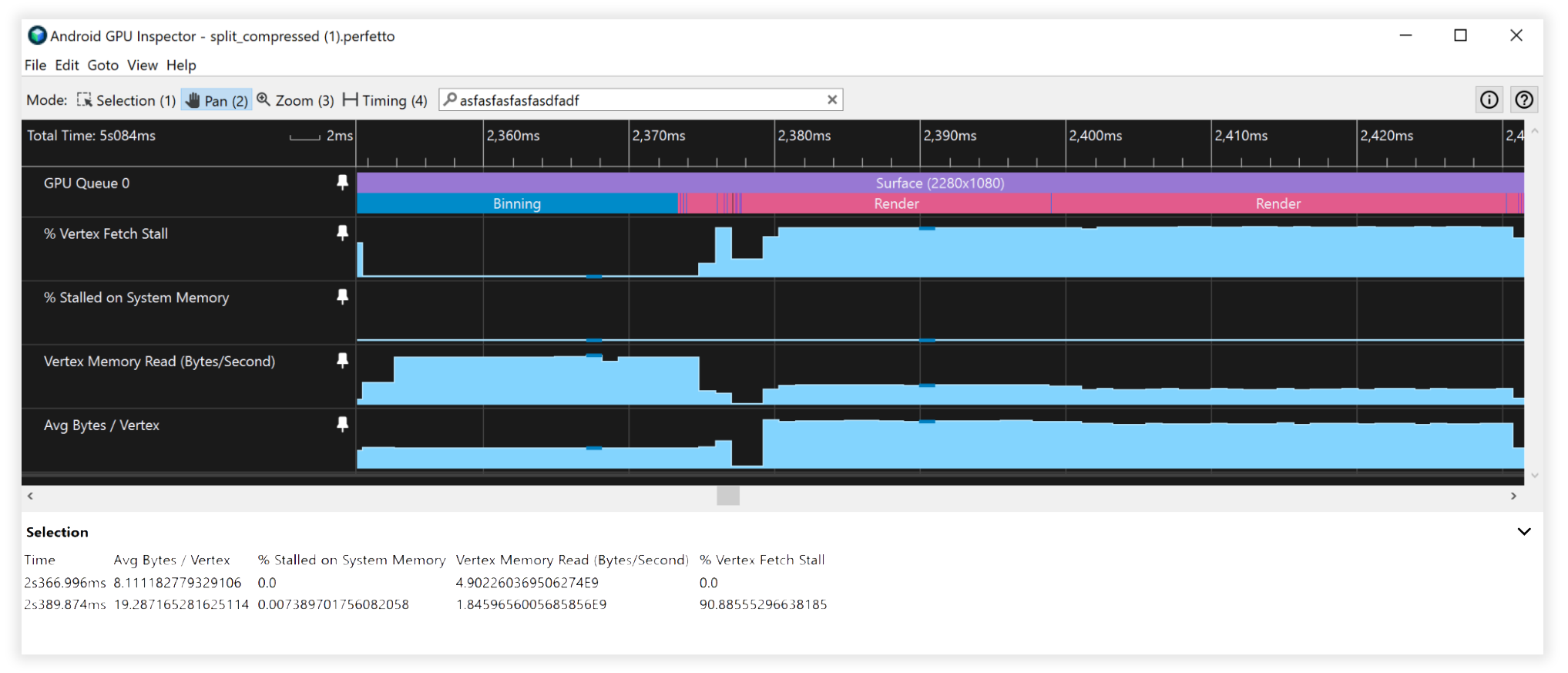## 其他注意事项

### 16 位与 32 位索引缓冲区数据

• 始终拆分网格/对网格进行分块，以便它们适配 16 位索引缓冲区（最多 65536 个唯一顶点）。这将有助于在移动设备上进行具有索引的渲染，因为提取顶点数据的成本更低，且功耗更少。

### 不受支持的顶点缓冲区属性格式

• SSCALED 顶点格式在移动设备上并未得到广泛支持，并且如果这些格式没有硬件支持，那么使用它们可能会严重影响尝试模拟它们的驱动程序的性能。始终选择使用 SNORM，并使用几乎可以忽略不计的 ALU 来进行解压缩。
[]
[]